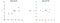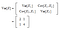# Principal Component AnalysisEl Capitan glows in the early morning light in Yosemite National Park, California (source)

There are many techniques to decompose features into a smaller subset. This can be useful for exploratory data analysis, visualization, making predictive models or clustering. In this tutorial, you will discover the Principal Component Analysis for dimensionality reduction.

The main idea of Principal Component Analysis (PCA) is to reduce the dimensionality of a data set consisting of many variables correlated with each other while retaining the variation present in the dataset, up to the maximum extent. It is a method that uses simple matrix operations from linear algebra and statistics to calculate a projection of the original data into the same number or fewer dimensions.

In an application, whether it is classification or regression, observation data that we believe contain information are taken as inputs and fed to the system for decision making. Ideally, we should not need feature selection or extraction as a separate process; the classifier or regressor should be able to use whichever features are necessary, discarding the irrelevant. However, there are several reasons why we are interested in reducing dimensionality as a separate preprocessing step:

• In most learning algorithms, the complexity depends on the number of input dimensions (d), as well as on the size of the data sample (N) and for reduced memory and computation, we are interested in reducing the dimensionality of the problem. Decreasing d also decreases the complexity of the inference algorithm during testing.
• When an input is decided to be unnecessary, we save the cost of extracting it.
• Simpler models are more robust on small datasets. Simpler models have less variance, that is, they vary less depending on the particulars of a sample, including noise, outliers, and so forth.
• When data can be explained with fewer features, we get a better idea about the process that underlies the data and this allows knowledge extraction.
• When data can be represented in a few dimensions without loss of information, it can be plotted and analyzed visually for structure and outliers.The left graph is our original data X; the right graph would be our transformed data(source)

There are two main methods for reducing dimensionality: feature selection and feature extraction.

• In feature selection, we are interested in finding k of the d dimensions that give us the most information and we discard the other (d−k) dimensions.
• In feature extraction, we are interested in finding a new set of k dimensions that are combinations of the original d dimensions. These methods may be supervised or unsupervised depending on whether or not they use the output information. The best known and most widely used feature extraction methods are Principal Components Analysis (PCA) and Linear Discriminant Analysis (LDA), which are both linear projection methods, unsupervised and supervised respectively.

Background Mathematics

I have included in my GitHub repo a section on Statistics which looks at distribution measurements, or, how the data is spread out. The other section is on Matrix Algebra and looks at eigenvectors and eigenvalues, important properties of matrices that are fundamental to PCA. You can reach my repo via using this link.

PCA is a way of recognizing patterns in data, and expressing the data in such a way as to highlight their similarities and variances. Since patterns in data can be hard to find in data of high dimension PCA is a powerful tool for analyzing data. The other main advantage of PCA is that once you have found these patterns in the data, and you compress the data by reducing the number of dimensions, without much loss of information.

Let us see an example to get some intuition: Assume we are given a class of customers with grades on six different features and we want to order these customers. That is, we want to project the data onto one dimension, such that the difference between the data points become most apparent. We can use PCA. The eigenvector with the highest eigenvalue is the direction that has the highest variance, that is, the direction on which the customers are most spread out. This works better than taking the average because we take into account correlations and differences in variances.

In practice we understand that some eigenvalues have little contribution to variance and may be discarded. Then, we take into account the leading k components that explain more than, for example, 95 percent, of the variance. When λi are sorted in descending order, the proportion of variance explained by the k principal components is

(λ 1 + λ 2 + … + λ k ) / (λ 1 + λ 2 + … + λ k+ .. + λ d)

If the dimensions are highly correlated, there will be a small number of eigenvectors with large eigenvalues and k will be much smaller than d and a large reduction in dimensionality may be attained. This is typically the case in many image and speech processing tasks where nearby inputs (in space or time) are highly correlated. If the dimensions are not correlated, k will be as large as d and there is no gain through PCA

Method

Let’s take a step-by-step look at the path we need to follow for PCA.

Step 1: Normalize the data

First step is to normalize the data that we have so that PCA works properly, you have to subtract the mean from each of the data dimensions. The mean subtracted is the average across each dimension. So, all the x values have xb (the mean of the x values of all the data points) subtracted, and all the y values have yb subtracted from them. This produces a data set whose mean is zero.

Step 2: Calculate the covariance matrix

This is done in exactly the same way as was discussed in my GitHub repo. Since the data is 2 dimensional, the covariance matrix will be 2x2. There are no surprises here, so I will just give you the result:Covariance Matrix

Step 3: Calculate the eigenvalues and eigenvectors

Next step is to calculate the eigenvalues and eigenvectors for the covariance matrix. The same is possible because it is a square matrix. ƛ is an eigenvalue for a matrix A if it is a solution of the characteristic equation:

det(Α-λ I)=0

Where, I is the identity matrix of the same dimension as A which is a required condition for the matrix subtraction as well in this case and det is the determinant of the matrix. For each eigenvalue ƛ, a corresponding eigen-vector v, can be found by solving:

Αν=λν

Step 4: Choosing components and forming a feature vector:

We order the eigenvalues from largest to smallest so that it gives us the components in order or significance. Here comes the dimensionality reduction part. If we have a dataset with n variables, then we have the corresponding n eigenvalues and eigenvectors. It turns out that the eigenvector corresponding to the highest eigenvalue is the principal component of the dataset and it is our call as to how many eigenvalues we choose to proceed our analysis with. To reduce the dimensions, we choose the first p eigenvalues and ignore the rest. We do lose out some information in the process, but if the eigenvalues are small, we do not lose much.

Next, we form a feature vector which is a matrix of vectors, in our case, the eigenvectors. In fact, only those eigenvectors which we want to proceed with. Since we just have 2 dimensions in the running example, we can either choose the one corresponding to the greater eigenvalue or simply take both.

Feature Vector = (eig1, eig2)

Step 5: Deriving the New Data Set

This is the final step where we actually form the principal components using all the math we did till here. For the same, we take the transpose of the feature vector and left-multiply it with the transpose of scaled version of original dataset.

New Data = Feature Vector^T x Scaled Data^T

Here,

• New Data is the Matrix consisting of the principal components,
• Feature Vector is the matrix we formed using the eigenvectors we chose to keep,
• Scaled Data is the scaled version of original dataset.

All the eigenvectors of a matrix are perpendicular to each other. So, in PCA, what we do is represent or transform the original dataset using these orthogonal (perpendicular) eigenvectors instead of representing on normal x and y axes. We have now classified our data points as a combination of contributions from both x and y. The difference lies when we actually disregard one or many eigenvectors, hence, reducing the dimension of the dataset. Otherwise, in case, we take all the eigenvectors in account, we are just transforming the co-ordinates and hence, not serving the purpose.

Conclusion

I hope you found this article helpful! Check out some of the resources below for more in-depth discussions of PCA. Let me know what you think, especially if there are suggestions for improvement.

References

 Alpaydın, E. 2010. “Introduction to Machine Learning.”The MIT Press Cambridge, Massachusetts, London, England

 Smith, L. 2002. “A Tutorial on Principal Components Analysis”

Data Scientist